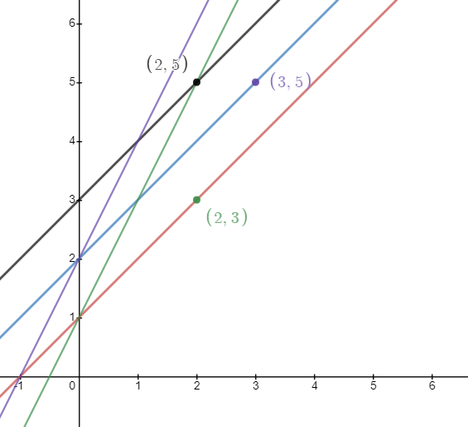# Perfect PointsYouKnowWhoContest Based on SUST Int...
Limits 4s, 512 MB

Perfect lines are the lines y=mx+c such that m and c are positive integers.

Perfect points are the points (x, y) such that it is on a Perfect line y=mx+c and x-c=1.

The following figure illustrates the first few Perfect lines and Perfect points
:Given an integer K, you need to find the Kth smallest positive integer y such that there exists a Perfect point having Y-coordinate equals to y.

## Input

The first line will contain an integer t(1 ≤ t ≤ 20), the number of test cases.

Each of the next t lines will contain an integer K(1 ≤ K ≤ 109).

## Output

Output the Kth smallest positive integer y such that there exists a Perfect point having Y-coordinate equals to y. We can show that the answer doesn't exceed 1018.

## Sample

InputOutput
3
1
2
3

3
5
7NumberTheoryuDebug

### Submit